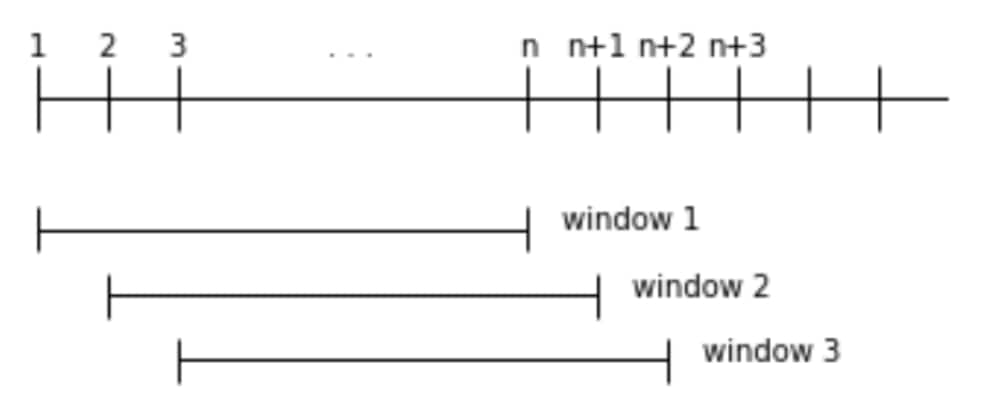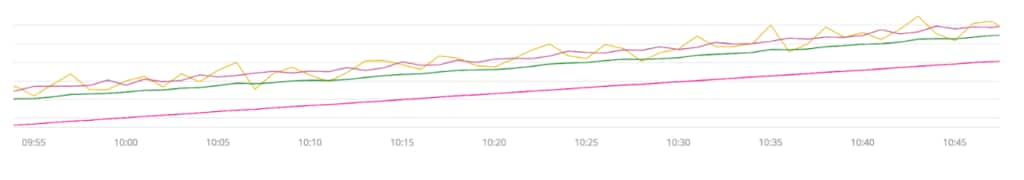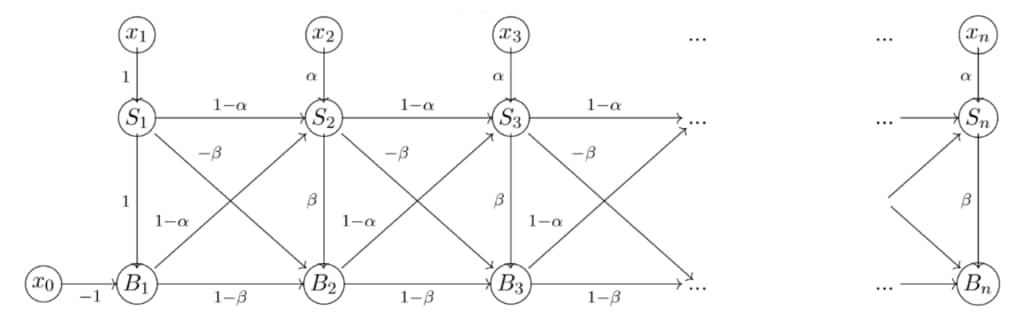DEVOPS

# Incremental-Decremental Methods for Time Series Analysis

Note: This is an extended abstract of a research-track talk given by Joe at the Open Data Science Conference, East 2018. An article on the double exponential smoothing algorithm has been accepted for publication in Communications in Statistics – Simulation and Computation.

Modern computing environments are instrumented to emit a wide variety of metrics, ranging from infrastructure metrics such as CPU, disk, memory, and network utilization — all the way to user experience, API, engagement, DevOps metrics, and business metrics.

Monitoring these metrics to detect and prevent service degradation and outages poses a variety of challenges to analyzing streaming data. In addition to the formidable engineering challenges involved in handling the scale and speed of monitoring machine data, there is a core algorithmic challenge: to develop extremely efficient yet high-quality methods for such time series tasks as anomaly detection, forecasting, and classification. As these environments are quite dynamic, the algorithms that operate on the data they produce must possess a mechanism for discarding stale data. A natural choice is the rolling window methodology: computing a statistic (or anomaly score, or forecast) on the most recent (fixed length) window of data, and updating the computation as new datapoints arrive and old datapoints “age out” of the window.This post discusses some rolling window algorithms developed here at SignalFx for processing metric time series at scale.

## Incremental-Decremental Methods

In the streaming setting, re-computing over the entire window upon every arrival is unacceptable for both performance and resource reasons. For a rolling window method to work, it is essential to develop an incremental-decremental method: this means datapoints can be added and removed efficiently, with the computation at each iteration and the overhead of the entire computation remaining constant (independent of the length of the rolling window).

The general strategy for incremental-decremental methods is to decompose the quantity of interest into pieces which can be easily updated. For example, to calculate a rolling variance, instead of maintaining the value of the variance itself, one maintains the count, sum, and sum of squares of the datapoints in the window, quantities which have straightforward update rules. Then, when the variance is requested, these quantities are assembled into the variance via the well-known formula (sum of squares / count) – (sum / count) * (sum / count). (This is correct in exact arithmetic. For numerical reasons, we prefer Welford’s method, explained in his 1962 Technometrics article “Note on a Method for Calculating Corrected Sums of Squares and Products.”)

We will discuss incremental-decremental methods for calculating double exponential smoothing and the Kwiatkowski–Phillips–Schmidt–Shin (KPSS) stationarity statistic on a rolling window. Our goal is to provide a glimpse into the underlying algorithms for calculating these quantities; for a discussion of use cases and SignalFlow examples, check out previous posts about double exponential smoothing and the KPSS statistic.

## Exponential Smoothing

Single exponential smoothing is a time series smoothing technique that places more weight on recent observations, and double exponential smoothing is a variant that models both the level and the trend of the time series. Assuming initializations for the level (whose value at time t is denoted St) and trend (denoted Bt) of the time series {xt}, double exponential smoothing updates iteratively as follows:

##### Bt =  β (St – St-1) + (1 –  β) Bt-1

Here and β are smoothing parameters between 0 and 1. Note that forecasts can be constructed via the formula St+c = St + c Bt. Compared to usual moving averages, exponentially weighted moving averages lag less; when a trend is present, the lag can be reduced even further by utilizing double exponential smoothing. In the following chart, the input signal is yellow. Going from bottom to top (most to least laggy), the moving average is pink, the exponentially weighted moving average is green, and the double exponentially weighted moving average is purple.Exponential smoothing models suppose old data becomes irrelevant; as the influence of a datapoint decays exponentially with time, these models typically operate with an infinite window. However, in a system supporting ad hoc queries and computations against a time series database with significant churn, for example due to ephemeral computing environments such as containers and functions, there is no canonical “start time” for a computation. It is therefore desirable for the results of the computation to be independent of the chosen start time, e.g., so a visualization is consistent as you pan across the chart displaying the smoothed time series. This also serves as a principled way to reduce computation size when a user may request, for example, the exponentially weighted moving average of a series for which there are several weeks of 1-second data available, amounting to 604,800 datapoints per week.

Using a rolling window eliminates the dependence on the start time, and we have devised incremental-decremental rolling window implementations of single and double exponential smoothing. While rolling window single exponential smoothing can be calculated along the same lines as traditional rolling window statistics (mean, variance, etc.), achieving the same for double exponential smoothing involves a novel representation of the computation as a weighted directed acyclic graph.The weights reflect the smoothing parameters and the graph structure encodes the arithmetic operations. Adding a datapoint appends some nodes and edges to the graph, and removing a datapoint alters the initial structure of the graph. The graph structure suggests the additional state required to efficiently remove datapoints from the computation, and maintaining the state amounts to propagating some quantities through the graph. The additional state reflects how the terminal vertices change with respect to the initial structure.

## KPSS Statistic

At the heart of many anomaly detection algorithms is a comparison between current and past values of a time series. The current period is declared anomalous when some statistic comparing these distributions is “too large.” In addition to a well-chosen statistic, this approach requires a careful selection of historical data against which to compare the present. For a stationary time series (i.e., one whose distribution does not change with time), it is natural to compare current values to recent history, whereas for time series with seasonality, it makes sense to compare against the same periods in previous seasons. Furthermore, understanding whether a series is stationary is an important step in many approaches to time series modeling.

The KPSS statistic, originally developed in econometrics for offline analysis of macroeconomic time series, tests the hypothesis that a series is stationary around a fixed level, or around a linear trend. It essentially consists of a sum of squared partial sums of residuals (from the mean, or from a regression line), appropriately normalized. The statistic can be used to guide the selection of an anomaly detection algorithm, or to evaluate whether a sequence of transformations (e.g., a seasonal differencing, or forming the ratio of two series) has produced a stationary series.

As a step towards streaming time series classification in the SignalFx platform, our incremental-decremental method of calculating the KPSS statistic makes it possible to perform these tasks in a fully streaming setting, and allows for an anomaly detection scheme to change dynamically with the characteristics of the time series. Expressing the KPSS statistic in terms of easily updatable pieces is accomplished by a series of delicate algebraic manipulations. Both the rolling mean and variance, for example, may be expressed as an easy-to-evaluate function of quantities which are easy to increment and decrement. In this case, by contrast, quite a few quantities must be tracked, and the update rule for one quantity may involve several of the other tracked quantities. For example, if we are maintaining the quantity x1 + 2 * x2 + … + n * xn on the window {x1, x2, …, xn}, then the rule for decrementing (removing x1 and re-indexing) involves the difference

##### (x1 + 2 * x2 + … + n * xn) – (x2 + 2 * x3 + … + (n-1) * xn) = x1 + x2 + … + xn,

which is itself the sum of the points in the window, before x1 has been removed. In general, the order in which the updates are performed matters, and a sort of hierarchy among the tracked quantities emerges. Along the way, we obtain an incremental-decremental form of linear regression of a time series against intercept and trend terms, which by itself can be used to form forecasts.

Incremental-decremental implementations of double exponential smoothing and the KPSS statistic expand the range of anomaly detection, forecasting, and time series classification that can be accomplished in a high-performance streaming environment.Posted by

#### Joe Ross

Joe is a data scientist at SignalFx. Previously Joe worked at other startups and in academia as a professor and researcher in mathematics.
TAGS
Show All Tags
Show Less Tags